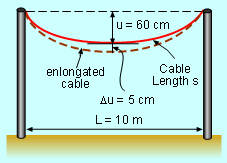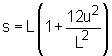Ch 3. Derivatives II Multimedia Engineering Math Higher OrderDerivatives RelatedRates Differential Newton'sMethod
 Chapter 1. Limits 2. Derivatives I 3. Derivatives II 4. Mean Value 5. Curve Sketching 6. Integrals 7. Inverse Functions 8. Integration Tech. 9. Integrate App. 10. Parametric Eqs. 11. Polar Coord. 12. Series Appendix Basic Math Units Search eBooks Dynamics Fluids Math Mechanics Statics Thermodynamics Author(s): Hengzhong Wen Chean Chin Ngo Meirong Huang Kurt Gramoll ©Kurt GramollMATHEMATICS - CASE STUDY IntroductionHanging Cable An electric cable company is replacing the old cable in Dallas. Without the supporting steel cable, the electric cable that hangs between two poles form an arc because of the gravity. In the summer, the cable sinks for about 5 cm due to temperature, how much does the cable elongate? What is known: The distance between the two poles, L, is 10 meters. The distance between the lowest point of the arc and the line of the top of the two poles, u, is 60 cm. The mathematics formula to calculate the length of the cable, s, isQuestions How much does the cable elongate when its lowest point goes down for 5 cm? Approach 1 meter equals 100 centimeters The cable length,s, is a differentiable function. Differential du is independent variable and the differential ds is defined in terms of du by ds = f '(u)du.